• 感知机模型 线性判别分析 高斯判别分析

感知机模型
线性判别分析
高斯判别分析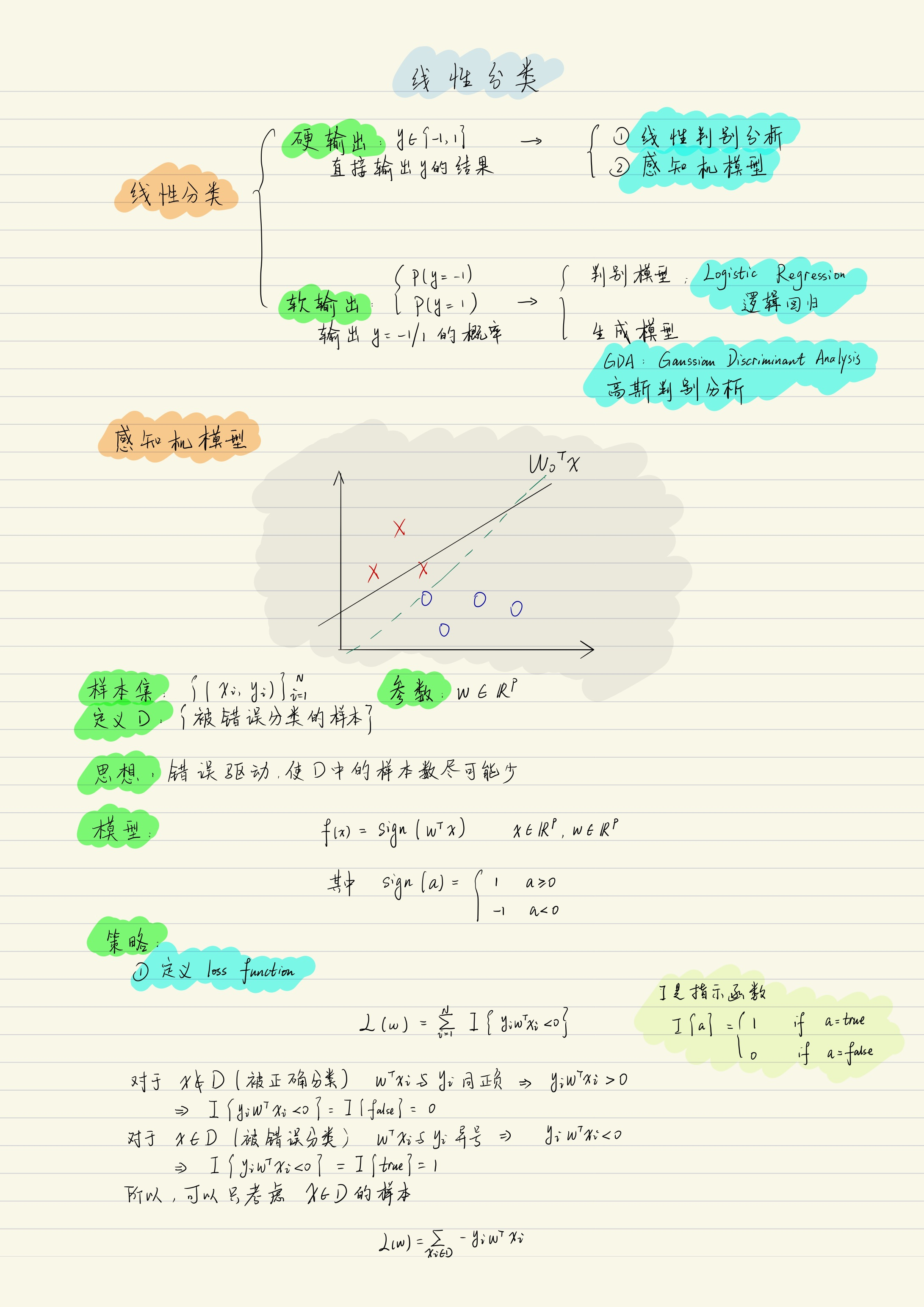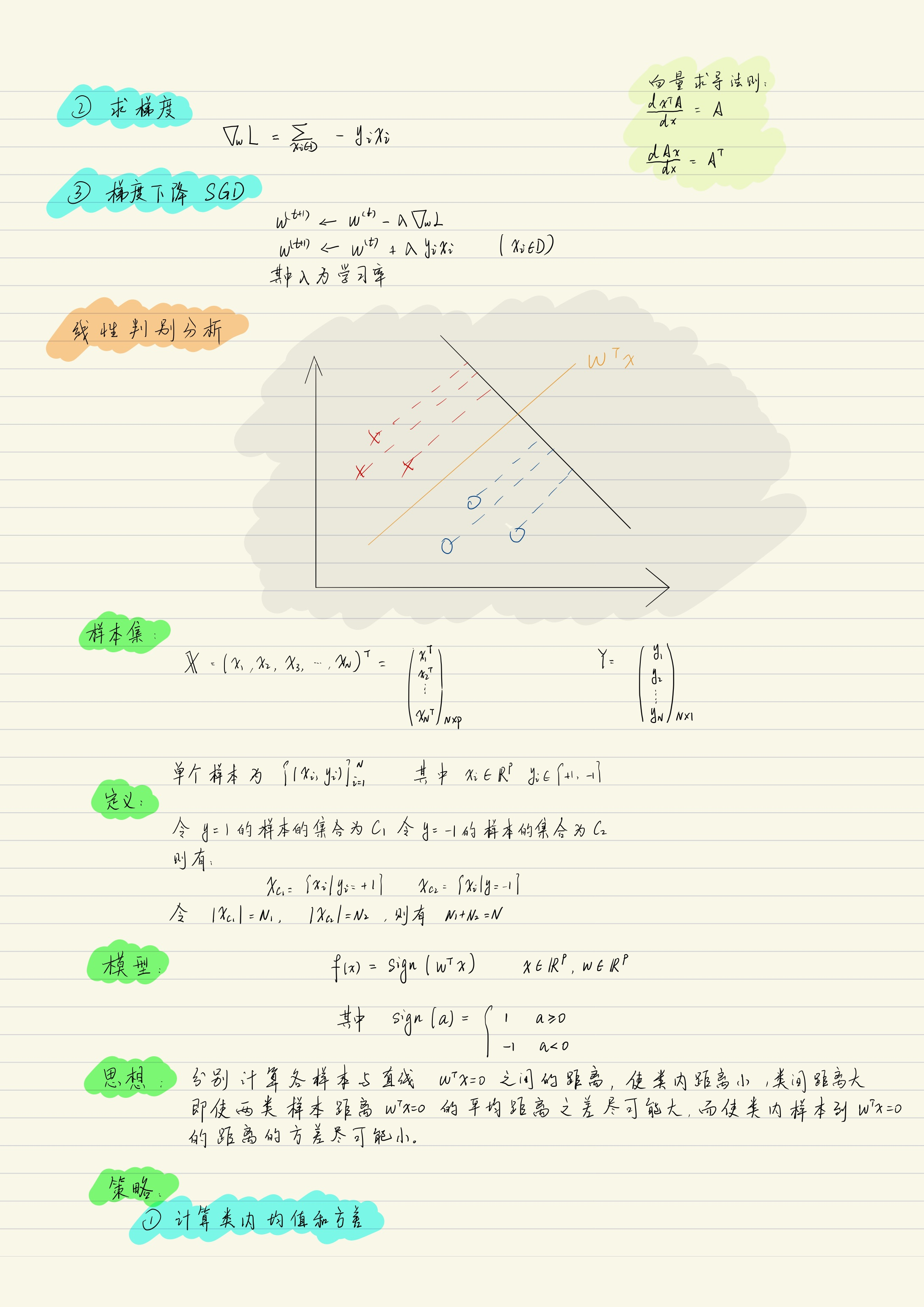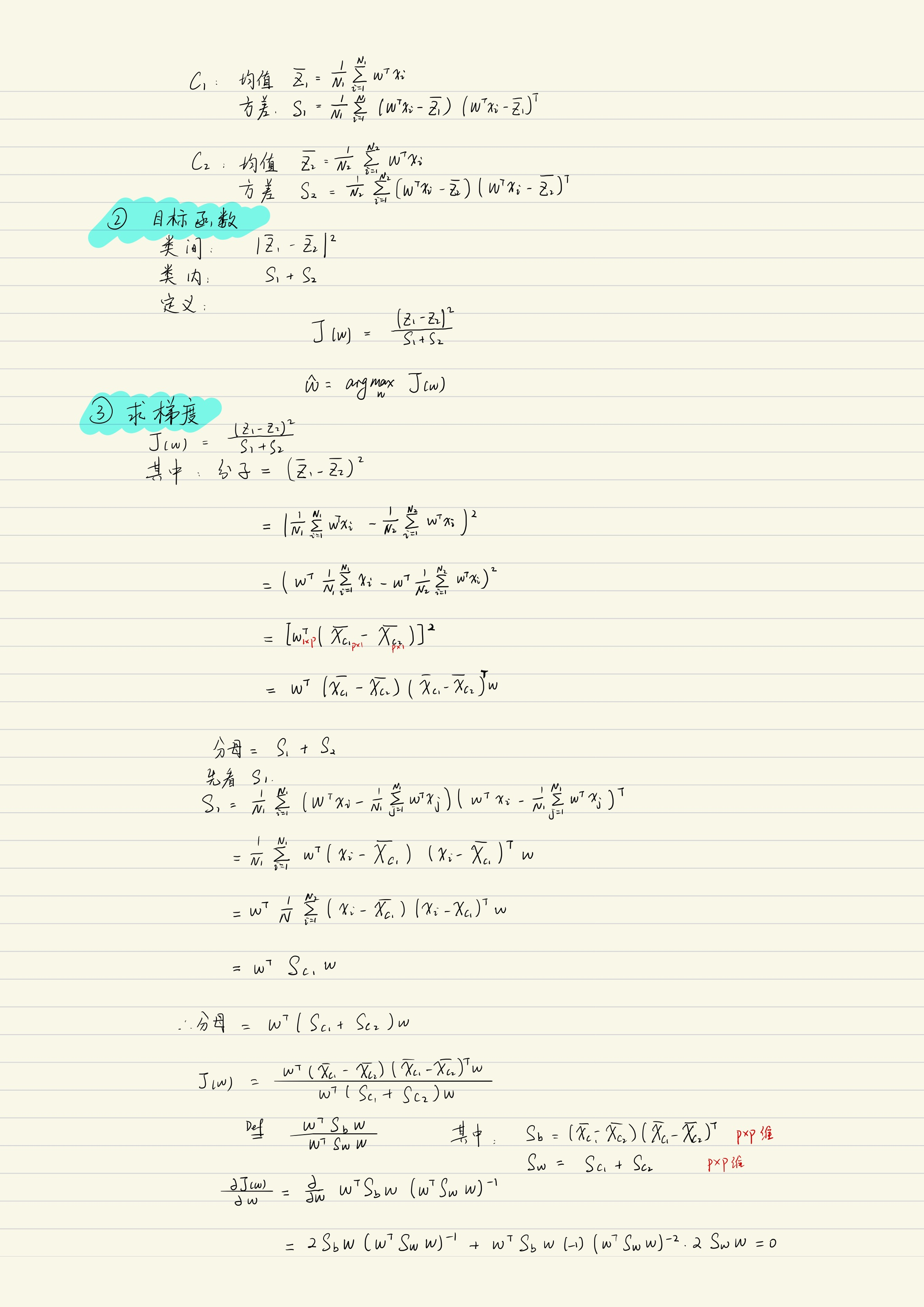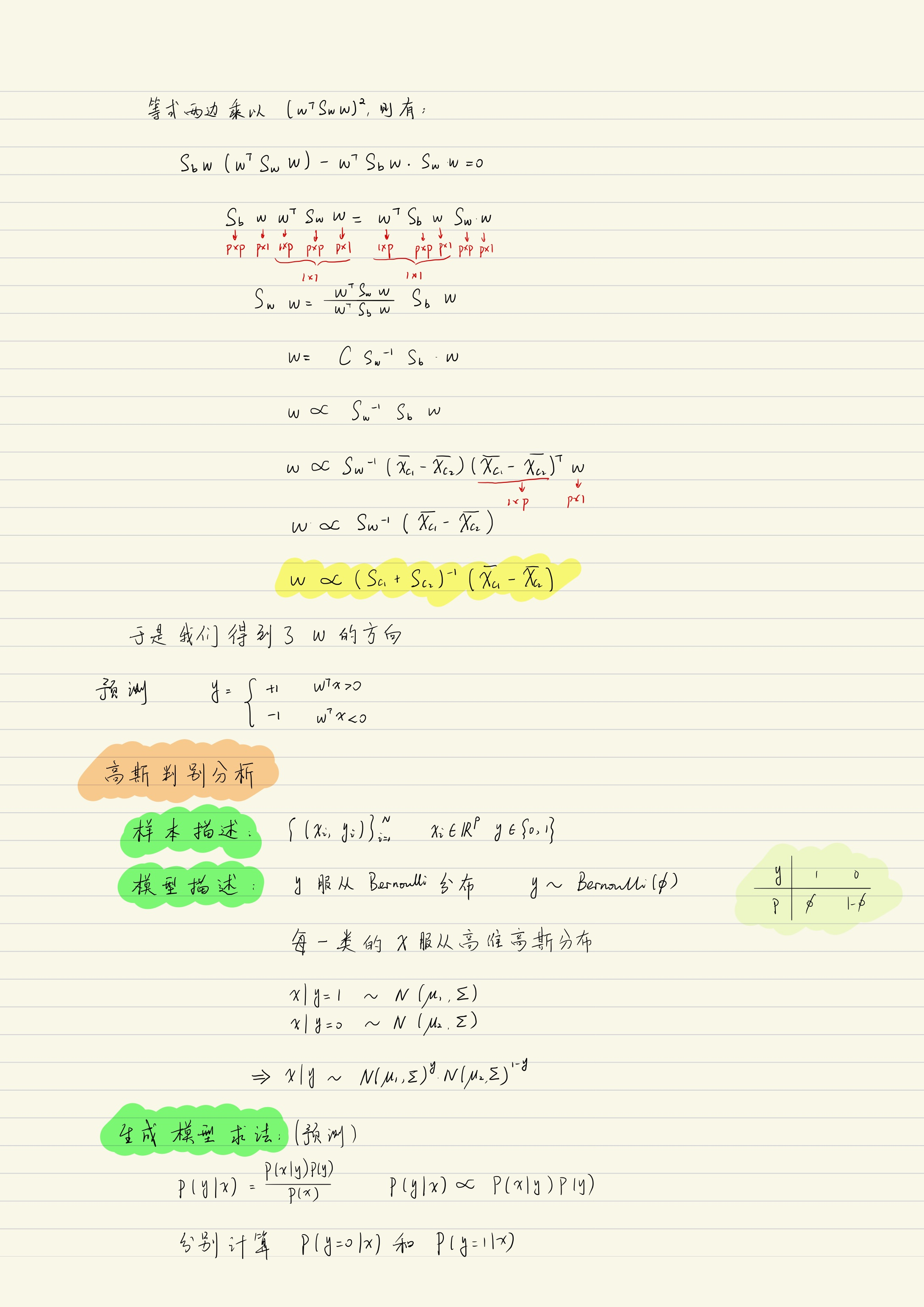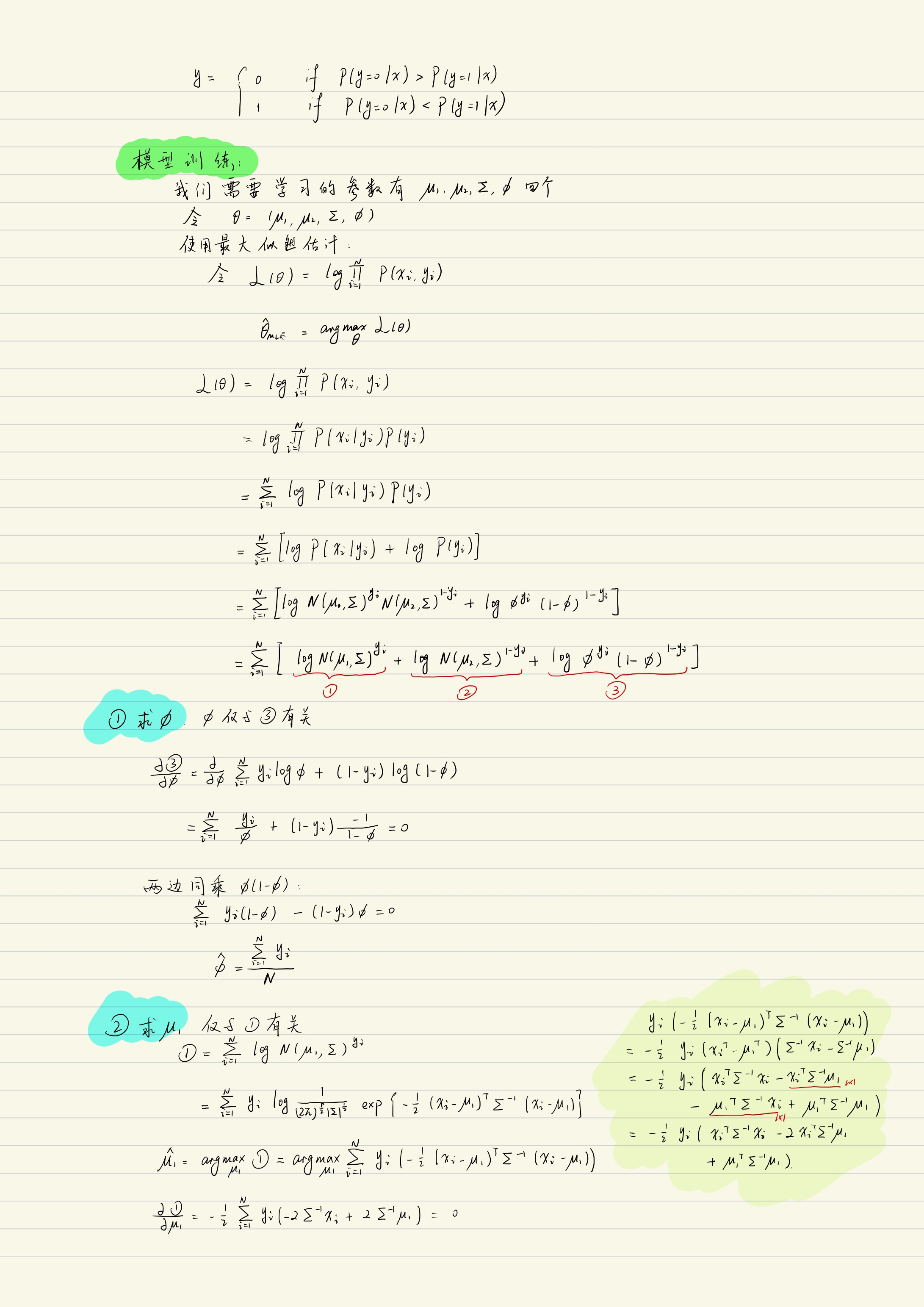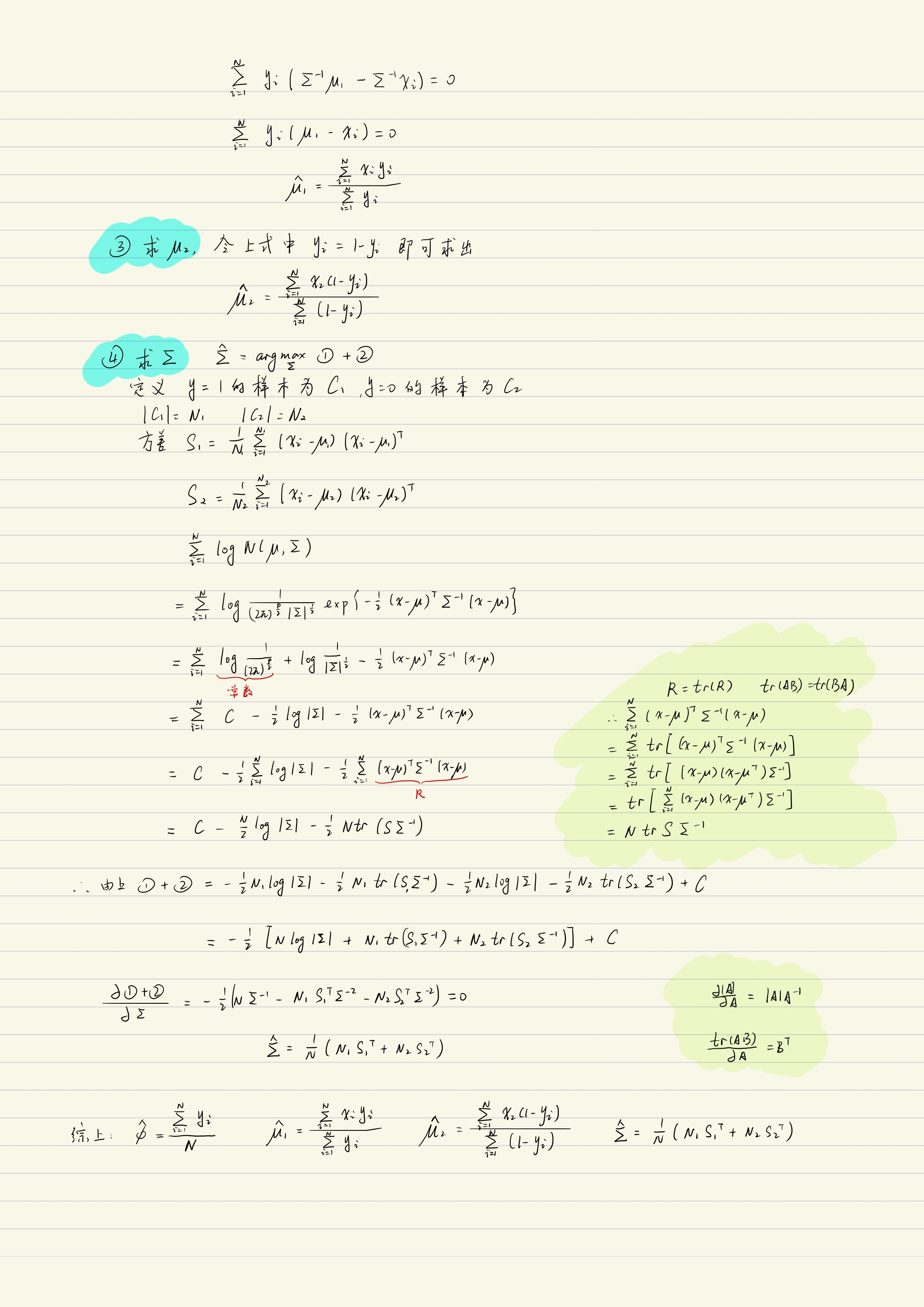展开全文线性分类 感知机
• ## 判别分析

千次阅读 2018-11-27 23:53:17
简要介绍了判别分析中的DISCRIM过程、STEPDISC过程和CANDISC过程。
根据已有的数据分类知识，建立一个判别准则，使其错判率最低，进而基于这个判别准则实现对未知样本所属类别进行判断。
一般判别分析
DISCRIM过程
格式：
proc discrim data=数据集名;
class 变量; *此语句必需的，指定判别分析用的分类变量名;
var 变量列表；*指定判别分析中使用的变量;
run;

注：
1.proc过程选项：
I.list：显示已知分类的数据集重分类的结果；
ii.testlist：在结果窗口中显示对检验未知样本的所有分类结果；
iii.testdata=待分析数据集名；
例
根据下面第一张表对经济发达水平的判别，对第二张表进行经济发达水平的判别分析。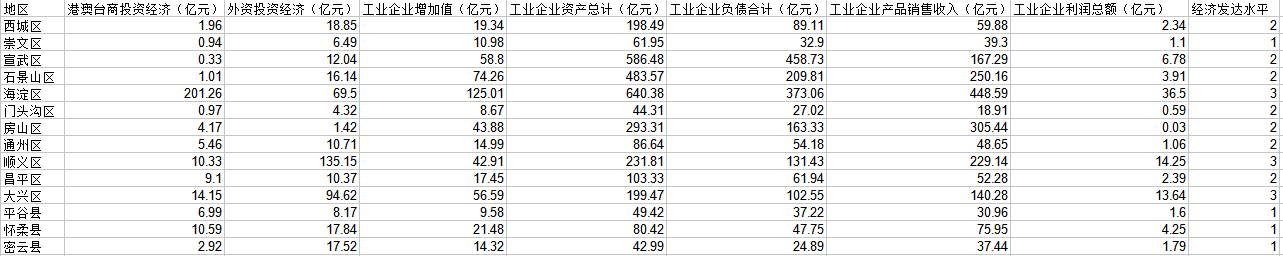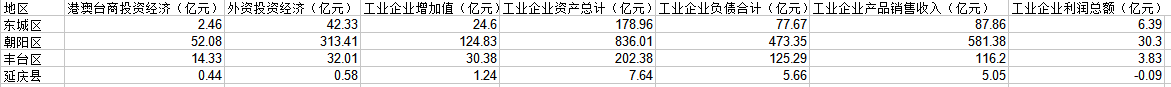代码：
data test1;        /*创建判别分析数据*/
input area$x1-x7 type; cards; 西城区 1.96 18.85 19.34 198.49 89.11 59.88 2.34 2 崇文区 0.94 6.49 10.98 61.95 32.9 39.3 1.1 1 宣武区 0.33 12.04 58.8 586.48 458.73 167.29 6.78 2 石景山区 1.01 16.14 74.26 483.57 209.81 250.16 3.91 2 海淀区 201.26 69.5 125.01 640.38 373.06 448.59 36.5 3 门头沟区 0.97 4.32 8.67 44.31 27.02 18.91 0.59 2 房山区 4.17 1.42 43.88 293.31 163.33 305.44 0.03 2 通州区 5.46 10.71 14.99 86.64 54.18 48.65 1.06 2 顺义区 10.33 135.15 42.91 231.81 131.43 229.14 14.25 3 昌平区 9.1 10.37 17.45 103.33 61.94 52.28 2.39 2 大兴区 14.15 94.62 56.59 199.47 102.55 140.28 13.64 3 平谷县 6.99 8.17 9.58 49.42 37.22 30.96 1.6 1 怀柔县 10.59 17.84 21.48 80.42 47.75 75.95 4.25 1 密云县 2.92 17.52 14.32 42.99 24.89 37.44 1.79 1 ; run; data test2; /*创建待判别分析数据*/ input area$ x1-x7;
cards;
东城区 2.46  42.33 24.6  178.96 77.67 87.86 6.39
朝阳区 52.08 313.41 124.83 836.01 473.35 581.38 30.3
丰台区 14.33 32.01 30.38 202.38 125.29 116.2 3.83
延庆县 0.44  0.58  1.24  7.64  5.66  5.05 - 0.09
;
run;
proc discrim data=test1 testdata=test2 list testlist;
class type;
var x1-x7;
run;

结果：
一些基本信息：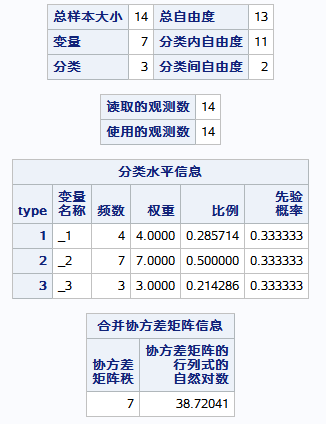以下是对已知分类的数据重分类后的结果：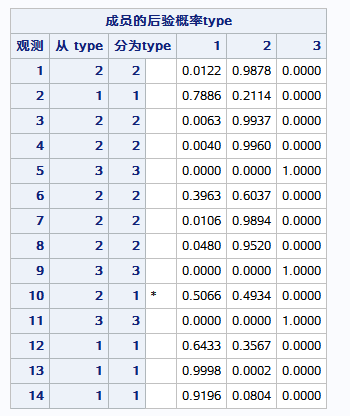以下是对未知分类数据进行分类的结果：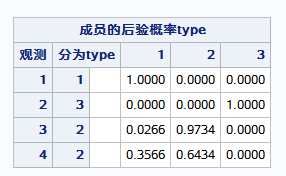典型判别分析
即通常的Fisher判别分析。
CANDISC过程
类似于主成分分析，通过数据降维，找一些变量，其为已存在变量的线性组合，使得依据这些变量可以很好地对数据进行分类判别。若要获得完整的判别分析结果，还需要将CANDISC过程的输出结果作为DISCRIM过程的输入，进行一般判别分析。
格式：
proc candisc data=数据集名 outstat=数据集名;
class 变量;
var 变量;
run;

注：
1.proc过程的选项：
a.outstat指定一个数据集，包含典型判别分析各种统计量；
逐步判别分析
思想类似于逐步回归分析
STEPDISC过程
用于在判别分析之前筛选出对数据的判别具有显著影响的变量，凭借这些结果（var var1 ... varm）再使用Discrim过程进行一般判别分析。
格式：
proc stepdisc data=数据集名;
class 分类变量;
var 指标变量; *逐步分析判别中使用的变量；

注：
1.proc stepdisc选项：
a.method：指定筛选变量的方法，包括forward、backward、stepwise；
例
根据下面第一张表对评价等级的判别，对第二张表进行经济发达水平的判别分析。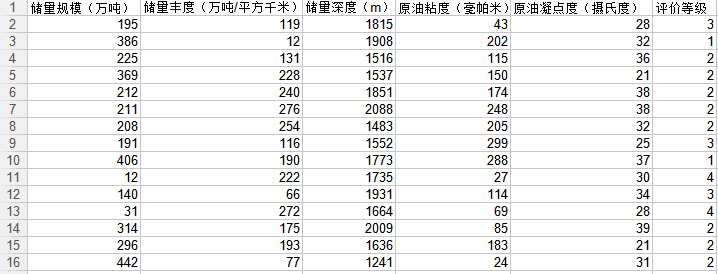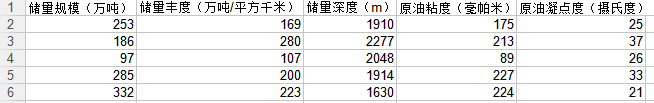代码：
data test;      /*创建判别分析数据*/
input x1-x5 type;
cards;
195 119 1815 43 28 3
386 12 1908 202 32 1
225 131 1516 115 36 2
369 228 1537 150 21 2
212 240 1851 174 38 2
211 276 2088 248 38 2
208 254 1483 205 32 2
191 116 1552 299 25 3
406 190 1773 288 37 1
12 222 1735 27 30 4
140 66 1931 114 34 3
31 272 1664 69 28 4
314 175 2009 85 39 2
296 193 1636 183 21 2
442 77 1241 24 31 2
;
run;
data test2;       /*创建待判别分析的数据集*/
input x1-x5;
cards;
253 169 1910 175 25
186 280 2277 213 37
97 107 2048 89 26
285 200 1914 227 33
332 223 1630 224 21
;
proc stepdisc data=test stepwise;
class type;
var x1-x5;
run;
proc discrim data=test testdata=test2 list testlist;
class type;
var x1 x2 x4;
run;

结果：
先进行stepdisc过程，结果显示x1、x2、x4选入的逐步回归模型：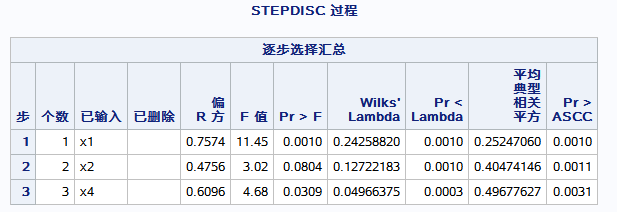以下是根据判别变量x1、x2、x4对未知数据进行分类的结果：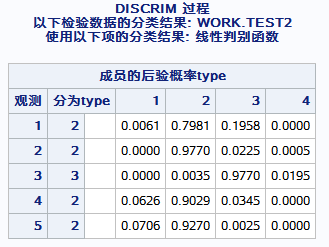展开全文SAS入门
• 从概率统计的角度来看，判别分析问题可归结为：设有个组（或或总体），所有组的样品都有相同的个指标，可表示为一个维向量，这组的分布函数为，均为维分布函数，对于给定的一个新样品，要求判断它属于哪个组。...
算法做了几年，尽然在博客上没留下什么脚印，主要写博客太麻烦了~，现在开始写点系列文章，以示点凭证~，今天写的是数据挖掘的基本定理及原理：分类原理之判别分析。

从概率统计的角度来看，判别分析问题可归结为：设有个组（或类或总体），所有组的样品都有相同的个指标，可表示为一个维向量，这组的分布函数为，均为维分布函数，对于给定的一个新样品，要求判断它属于哪个组。一般有距离判别、贝叶斯（Bayes）判别和费希尔（Fisher）判别
距离判别
马氏距离
在统计学中，特别是多元统计，欧氏距离有时不太合适，需使用马氏距离。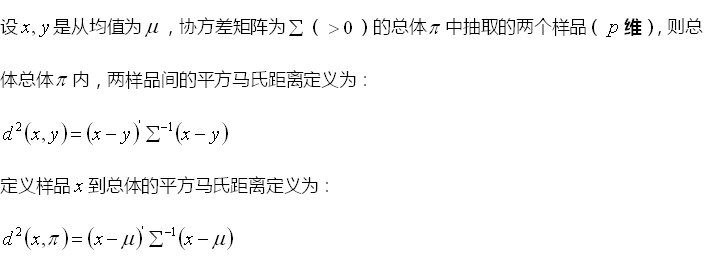贝叶斯判别

贝叶斯判别的引入：在两组距离判别中，如果属于组的样品个数比属于组的样品个数多得多，那只根据样品距离这两组的远近来判别其归属就有些不妥，即使比稍大些，人们往往倾向于判断属于组。因为判别之前他们已有了“先验”的认识，即来自组比来自组有更大的先验概率。先验概率可以根据组的大小、历史资料及经验等加以确定，常常带有一定的主观性。利用先验信息来进行判别是贝叶斯判别的一大特点。
是公式，所以只能用图片了~

展开全文多元统计 算法
• 线性回归线性分类硬输出-感知机硬输出-线性判别分析软输出-判别式：逻辑回归软输出-生成式：高斯判别分析软输出-生成式：朴素贝叶斯 线性分类 从线性回归到线性分类，通过激活函数或者降维来实现。 线性回归函数f...
线性回归线性分类硬输出-感知机硬输出-线性判别分析软输出-判别式：逻辑回归软输出-生成式：高斯判别分析软输出-生成式：朴素贝叶斯
线性分类
从线性回归到线性分类，通过激活函数或者降维来实现。
线性回归函数$f(w,b)=w^Tx+b$经过激活函数作用$y=f(w^Tx+b)$作用后变成硬分类问题$y\epsilon \left \{0,1 \right \}$或者变成软分类问题$y\epsilon [0,1],$函数$f$就称为激活函数，$f^{-1}$称为$link function,$
硬分类代表模型：感知机，线性判别分析
软分类代表模型：高斯判别分析(生成式模型)，逻辑回归(判别式模型)
硬输出-感知机
样本集：$\left \{ (x_i,y_i)\right \}_{i=1} ^N$
感知机思想：错误驱动。给定一个初始的分类平面$w^Tx，$再一步步移动这个平面使得被错误分类的点越来越少。被错误分类的集合用D表示
模型：$f(x)=sign(w^Tx),x\epsilon R^P,w\epsilon R^P,$其中当
$w^Tx \geq 0,sign(w^Tx)=1;w^Tx < 0,sign(w^Tx)=0$
策略定义为被错误分类的点的个数
策略：$loss function:L(w)=\sum_{i=1} ^N I\left \{ y_iw^Tx_i<0 \right \},$也就是当$y_iw^Tx_i<0$时样本是被错误分类的。
通俗点理解就是$w^Tx_i>0,y_i=1\rightarrow y_iw^Tx_i>0,$分类正确，反之分类错误。然而$L(w)$是不可导的，那么这个$loss function$就需要进行改进。虽然$L(w)$是不可导的，但是$y_iw^Tx_i$是可导的。
因此改进之后的$loss funtion$是这样：$L(w)=\sum_{x_i\epsilon D}-y_iw^Tx_i，$
对$w$求偏导：$\bigtriangledown _wL=-y_ix_i$
接下来算法只需用随机梯度下降法就能求出来了。
算法:SGD
$w^{(t+1)}\leftarrow w^{t}+\lambda \bigtriangledown _wL$
当然，上述的感知机算法都是基于数据线性可分为前提
硬输出-线性判别分析
样本集：$\left \{ (x_i,y_i)\right \}_{i=1} ^N,x_i\epsilon R^p,y_i\epsilon\left\{ c_1=1,c_2=-1 \right \}$
$x_{c_1}=\left \{x_i|y_i=+1 \right \},x_{c_2}=\left \{x_i|y_i=-1 \right \}$
令集合$x_{c_1}=N_1,x_{c_2}=N_2,$所以$N_1+N_2=N$
思想：类内小，类间大
如图所示：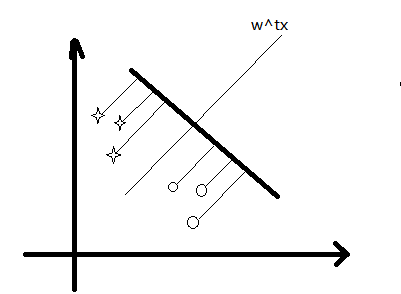同类的数据间隔尽量小(类内方差尽量小)，而不同类数据星星和圈圈之间的距离尽量大(类间大)。
我们投影的方向是$w,$也就是超平面对应的法向量。
点到平面的投影的线为$z，z_i=w^Tx_i$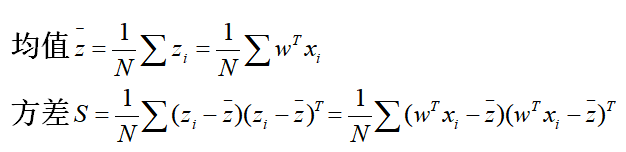所以不同类的均值可以表示出来：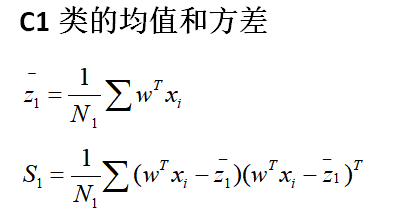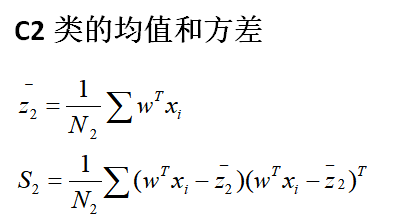那么类间的距离就可以用$(\bar z_1-\bar z_2)^2$表示；
类内的距离就用$S_1+S_2$表示。
那么为了达到我们的目的，也就是类内小，类间大，目标函数可以定义如下：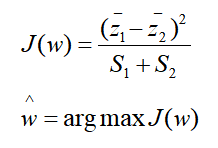令：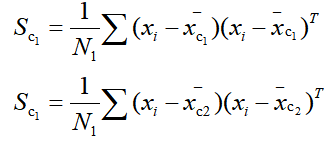化简我们的$J(w)$表达式，可得：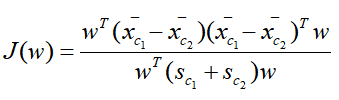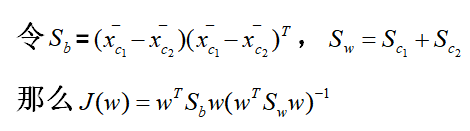这里的$S_b$就是类间方差，$S_w$是类内方差。
对w求偏导，令$\frac{\partial J(w)}{\partial w}=0，$求得：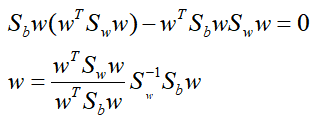由于我们只关心$w$的方向而不管其大小，那么$w \propto S_w^{-1}(\bar x_{c_1}-\bar x_{c_2})$；如果$S_w^{-1}$是个对角矩阵或者单位矩阵，那么$w \propto (\bar x_{c_1}-\bar x_{c_2})$
软输出-判别式：逻辑回归
样本集：$\left \{ (x_i,y_i)\right \}_{i=1} ^N,x_i\epsilon R^p,y_i\epsilon\left\{ 0,1 \right \}$
引入sigmoid function：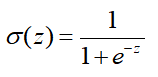其函数图像如下：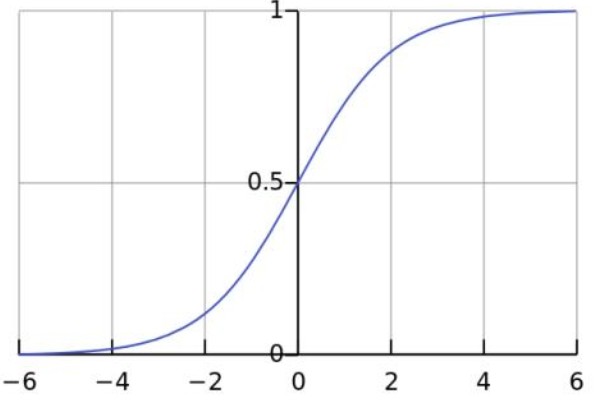代入有：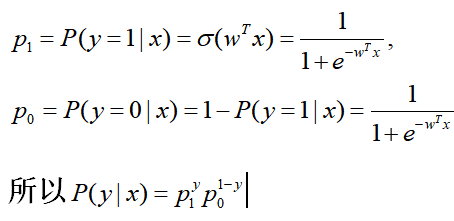接下来用极大似然估计求解$\hat{w}=\underset w {argmax}logP(Y|X)$就可以了。
软输出-生成式：高斯判别分析
样本集：$\left \{ (x_i,y_i)\right \}_{i=1} ^N,x_i\epsilon R^p,y_i\epsilon\left\{ 0,1 \right \}$
我们知道对于概率判别模型，我们可以直接求y值进行分类；而概率生成模型则是通过比较y=0和y=1的大小进行判断，根据贝叶斯公式$P(y|x)=\frac{P(x|y)P(y)}{P(x)}$我们知道，概率生成模型和分母项$P(x)$无关，所以可以对联合概率建模$P(y|x)\propto P(x|y)P(y)，$也就是后验=似然*先验。
模型：$\hat y=\underset{y\epsilon\left \{0,1\right\}}{argmax}P(y|x)=\underset y {argmax}P(y)P(x|y)$
那么y是服从伯努利分布$P(y)=\phi^y(1-\phi)^{1-y}$，
x服从于高斯分布,$x|y=1 \sim N(\mu_1,\varepsilon )^y$,$\left \{x_i|y_i=1,i=1,...,N\right \}$,此集合作为$c_1$,集合内元素的总个数为$N_1$，对应的方差为$S_1$。
$x|y=0 \sim N(\mu_2,\varepsilon )^y$,$\left \{x_i|y_i=0,i=1,...,N\right \}$,集合作为$c_2$,集合内元素的总个数为$N_2$，对应的方差为$S_2$。
$P(x_i|y_i)=N(\mu_1,\varepsilon )^y*N(\mu_2,\varepsilon )^{1-y}$
对数似然就能写成如下公式：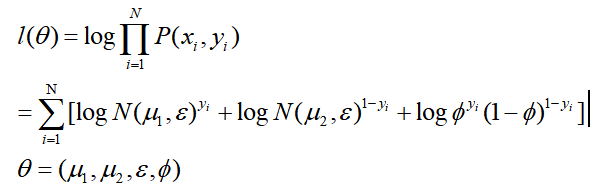求得$\hat \phi=\frac{N_1}{N},$
$\hat\varepsilon=\frac{1}{N}(N_1S_!+N_2S_2)$
$\hat\mu_1=\frac{\sum{i=1}{N}y_ix_i}{N_1}$
$\hat\mu_2=\frac{\sum{i=1}{N}y_ix_i}{N_2}$
软输出-生成式：朴素贝叶斯
样本集：$\left \{ (x_i,y_i)\right \}_{i=1} ^N,x_i\epsilon R^p,y_i\epsilon\left\{ 0,1 \right \}$
思想：朴素贝叶斯假设，也叫条件独立性假设。
朴素贝叶斯是最简单的概率图(有向图)模型。
条件独立性假设如图所示: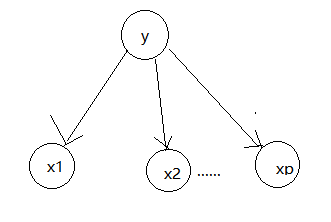也就是给定y的情况下，x1和x2相互独立。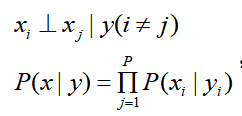做这个假设的目的就是为了简化运算，考虑高维情况。
朴素贝叶斯的目的也是给定x和y，判断其属于0还是1。
模型：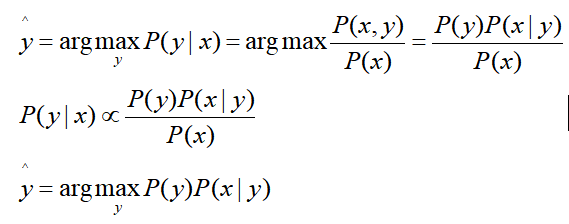对于P(y)，如果是二分类问题，其属于伯努利分布；如果是多分类问题，其属于categrial分布。
对于P(x|y),假定其遵循条件独立性假设。如果x是离散的，其属于categorical分布；如果x是连续的，其属于高斯分布。
求解可以直接用极大似然估计求解出来，在此就不做过多介绍了。


展开全文逻辑回归 感知机算法 朴素贝叶斯
• ## 判别分析基础

千次阅读 2016-03-29 12:46:57
判别分析与聚类分析不同,判别分析是在已知研究对象分成若干类型(或组别)并已取得各种类型的一批已知样品的观测数据。在实际中判别分析和聚类分析往往联合起来用，当总体分类不清楚时,可先用聚类分析对原来的一批样品...
• 判别分析包括可用于分类和降维的方法。线性判别分析（LDA）特别受欢迎，因为它既是分类器又是降维技术。二次判别分析（QDA）是LDA的变体，允许数据的非线性分离。最后，正则化判别分析（RDA）是LDA和QDA之间的折衷。...
• 一、聚类分析/判别分析 二、分类数的确定原则 三、Matlab系统聚类 四、K-均值聚类（K-means聚类） 五、Matlab判别分析 1.距离判别法 2.贝叶斯判别 一、聚类分析/判别分析 聚类分析就是把没有分类信息的资料...Matlab 聚类分析
• 因此，在多数数据分析的教材中，这两章是一前一后出现的，简而言之，聚类分析，其实是判别分析的基础，即在聚类分析的基础上，总结出各类的权值，将待判样本与各类权值做对比，距离最近的，即为一。 以下，给出我...
• spss进行判别分析步骤_spss判别分析结果解释_spss判别分析案例详解 1．Discriminant Analysis判别分析主对话框 如图 1-1 所示 图 1-1 Discriminant Analysis 主对话框 （1）选择分类变量及其范围 在主对话框中...
• 线性判别分析（Linear Discriminant Analysis） 线性判别分析Linear Discriminant Analysis 线性分类器 判别式函数discriminant functions从判别式或后验概率到决策面 线性判别分析Linear ...
• 判别分析包括可用于分类和降维的方法。线性判别分析（LDA）特别受欢迎，因为它既是分类器又是降维技术。二次判别分析（QDA）是LDA的变体，允许数据的非线性分离。最后，正则化判别分析（RDA）是LDA和QDA之间的折衷。...
• 上一篇写过线性判别分析处理二分类问题https://blog.csdn.net/z962013489/article/details/79871789，当使用LDA处理分类问题时，通常是作为一个降维工具来使用的。若我们有一个D维的样本集，该样本集包含C个类别共...python LDA 机器学习 降维 分类
• 本文使用LDA作为分类器在matlab下做实验。...　其中投影转换矩阵W按照LDA的经典理论生成，如下的LDA函数，并返回各个的投影后的（ｋ－１）维的均值。 LDA.m代码如下： function [W,center
• Fisher判别分析 将高维度空间的样本投影到低维空间上，使得投影后的样本数据在新的子空间上有最小的内距离以及最大的间距离，使得在该子空间上有最佳的可分离性 可以看出右侧投影后具有更好的可分离性。 ...计算机视觉
• ## R语言 判别分析

千次阅读 2016-12-27 10:48:29
#判别分析 用以判别个体所属群体的一种统计方法 判别分析重点是两群体的判别方法 #主要判别分析方法 有距离判别 贝叶斯判别 费歇判别法 1、关键点： #贝叶斯判别 贝叶斯判别式假定对研究对象已有一定的认识...
• 一、两分类问题 1、LDA分类 2、贝叶斯分类 三、分类问题
• 参考薛毅的《统计建模与R》里的判别分析，按他书里的例子用iris数据可以运行，我换了自己的X和G就不可以了，不明白问题出在哪里？ 代码和运行错误的截图如下： X1、G1是薛毅书里的数据，X、G是换我自己的数据之后...r语言
• 一年前就研究过Fisher线性判别分析，到...主要用于二分类问题，对于多类问题则可以次运用该方法就可以了； 2、Fisher线性判别分析的主要原理是将带有类别标签的高维样本投影到一个向量w（一维空间）上，使得在该向LDA详解
• 判别分析 判别分析是一种有效的对个案进行分类分析的方法。和聚类分析不同的是，...聚类分析分成几后，即可以作为判别分析的类别输入，进行判断。判别分析的模型按照判别的不同准则可以分为典型判别分析、贝叶...
• 鸢尾花数据的聚类分析和判别分析 摘要：本文基于鸢尾花数据的公开数据集，根据鸢尾花的萼片长度和宽度，花瓣的长度和宽度，对鸢尾花的类别归属进行聚类分析和判别分析。本文使用 R 语言，对鸢尾花的种类进行了聚类...分类
• 判别分析系列博文： 判别分析 （ distinguish analysis）(一)：距离判别 判别分析 （ distinguish analysis）(二)：Fisher 判别 判别分析 （ distinguish analysis）(三)：Bayes 判别 判别分析 （ distinguish ...
• 线性判别分析LDA 前言：我在我的第一家公司分析宏基因组数据时，碰到过LDA，不过当时没有去搞明白，今天有机会再来学习它。在这里，我们将了解到线性判别分析是属于一种线性分类器。 线性分类器是最简单的分类器。...
• 目录1. 简单的判别分析_ 距离判别法2. Fisher判别分析/线性判别分析 1. 简单的判别分析_ 距离判别法 2. Fisher判别分析/线性判别分析
• 文章目录判别分析判别分析的基本思想距离判别两总体情况总体情况贝叶斯判别费歇判别逐步判别 判别分析 本章介绍的判别分析适用于被解释变量是非度量变量的情形。在这种情况下，人们对于预测和解释影响一个对象...距离判别 多元统计
• ## R语言判别分析

万次阅读 多人点赞 2017-02-27 17:07:54
部分参考薛毅的《统计建模与R软件》和《R语言实战》本文中分三个方法介绍判别分析，Bayes判别，距离判别，Fisher判别。前两种判别方法都要考虑两个、或个总体协方差（这里是算方差，方差是协方差的一种）相等或...数据分析 r语言...